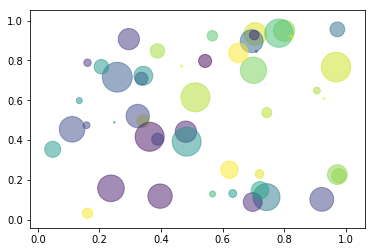# gist

 Pay Notebook Creator: Michelle Chung 0 Set Container: Numerical CPU with TINY Memory for 10 Minutes 0 Total 0
In :
#CrossCompute

outcome_select = """

Dropped Out - % of cohort
Total Regents - % of cohort
"""
attribute_select = """
Diversity Index

Diversity Index
economic_need_index
students_with_disabilities_1
"""
target_folder = '/tmp'

In :
import numpy as np
import matplotlib.pyplot as plt
%matplotlib inline

# Fixing random state for reproducibility
np.random.seed(19680801)

#N = 50
#x = np.random.rand(N)
#y = np.random.rand(N)
colors = np.random.rand(N)
area = (30 * np.random.rand(N))**2  # 0 to 15 point radii

axes = plt.scatter(x, y, s=area, c=colors, alpha=0.5)
plt.show()In :
# Save file to target folder to include it in the result download
target_path = target_folder + '/c.png'
figure = axes.get_figure()
figure.savefig(target_path)
print(f'c_image_path = {target_path}')

c_image_path = /tmp/c.png

In :
url = 'https://data.cityofnewyork.us/api/geospatial/r8nu-ymqj?method=export&format=Shapefile'

In :
import geotable

In :
dist_table = geotable.load(url)

In :
dist_table.iloc

Out:
school_dis                                                        16
shape_area                                               4.67636e+07
shape_leng                                                   35848.9
geometry_object    POLYGON ((-73.93311862859143 40.69579115384632...
geometry_layer       geo_export_bc9026ef-014b-4286-85ce-202961c6ce24
geometry_proj4                   +proj=longlat +ellps=WGS84 +no_defs
Name: 0, dtype: object